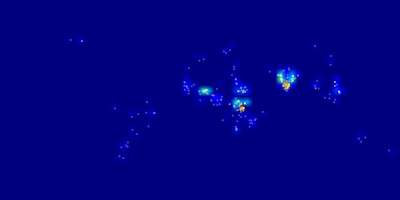## Wednesday, November 7, 2012

### Heat maps with Python

Heat maps are a quite often discussed topic on gis.stackexchange.com, probably because they are easy to understand and very appealing when showing spatial distributions of features. Working with a Python web framework, I looked for a Python way to create heat maps. Finally I came up with a script that uses OGR to read an input Shapefile and matplotlib to do the actual work.

Snippets from the script:

Read the input layer using OGR. Of course it can be in any format, that is supported by OGR.
```# get the shape file driver
shpDriver = osgeo.ogr.GetDriverByName('ESRI Shapefile')

# Open shape file with points
shpFile = 'activities.shp'
dataSource = shpDriver.Open(shpFile, 0)
if dataSource is None:
print 'Could not open file ' + shpFile
sys.exit(1)
# Get the layer
layer = dataSource.GetLayer()```

A bounding box and the number of cells need to be defined to calculate the resolution.
```# The global bounding box
xmin = -180.0
ymin = -90.0
xmax = 180.0
ymax = 90.0

# Number of columns and rows
nbrColumns = 72.0
nbrRows = 36.0

# Caculate the cell size in x and y direction
csx = (xmax - xmin) / nbrColumns
csy = (ymax - ymin) / nbrRows```

Knowing the cell size the script loops the whole extent and counts the number of feature in each cell. The number of features is stored in a two-dimensional array.
```rows = []
i = ymax
while i > ymin:
j = xmin
cols = []
while j < xmax:
# Set a spatial filter
layer.SetSpatialFilterRect(j, (i-csy), (j+csx), i)
# And count the features
cols.append(layer.GetFeatureCount())
j += csx
rows.append(cols)
i -= csy```
After preparing the data it's time for plotting this data with matplotlib. First a new figure is instantiated and the size is set. Then a new Axes object is created and added to the figure.
```# Create a new figure
import matplotlib.pyplot as plt
fig = plt.figure()
# Set the size
fig.set_size_inches((outputWidth / 100.0), ((outputWidth / 2.0) / 100.0))

ax = plt.Axes(fig, [0., 0., 1., 1.])
ax.set_axis_off()

Finally the array containing the number of features per cell is plotted using the imshow method. The result is saved to an image.
```# Create the heatmap
ax.imshow(rows, extent=[xmin, xmax, ymin, ymax], interpolation='nearest')
# Write the image to a file
plt.savefig('%s.png' % outputName)```

Optionally a world file can be written to georeference this result and use it in a GIS.
```# Write the world file to georeference the image
worldFile = open('%s.wld' % outputName, 'w')

# Pixel size in x direction
psx = (xmax - xmin) / outputWidth
worldFile.write('%s\n' % psx)
worldFile.write('0\n')
worldFile.write('0\n')

# Pixel size in y direction
pixelSizeY = (ymin - ymax) / (outputWidth / 2)
worldFile.write('%s\n' % pixelSizeY)

# Origin i.e. upper left corner
worldFile.write('%s\n' % (xmin + psx/2))
worldFile.write('%s\n' % (ymax + pixelSizeY/2))```

Using data from the Land Matrix database, an online public database that collects and visualize data on international land deals, I got the following results:A heat map showing world wide land deals
Instead of the nearest, a bilinear interpolation can be used to get a fuzzier heat map. With some more matplotlib functions, features can also be plotted on top.Plotted land deals on top of a bilinear interpolated heat map
Last but not least the georeferenced map can be used for further mapping or analysis in a GIS.A heat map showing land deals with country borders from Natural Earth
To me matplotlib seems a very powerful and comprehensive plotting library, and of course it offers a lot more options than I could show here.